## Introduction and Statistical Features of Data

I have been assigned the time series measuring turnover in Victorian supermarkets and grocery stores, measured in \$ millions. Before undertaking any statistical analysis, let’s visually examine a plot of the data.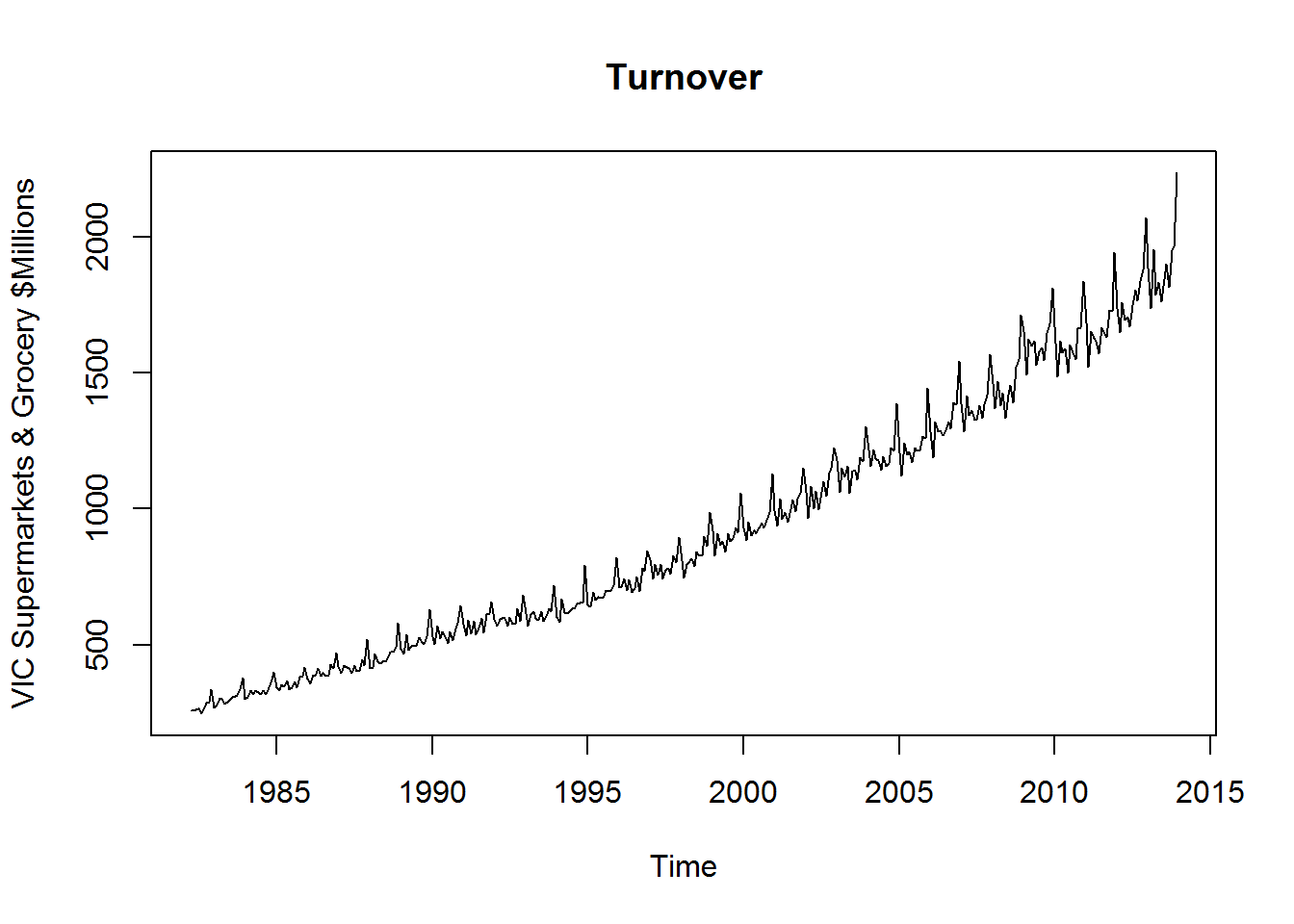Most significantly, it features a strong positive trend, and exhibits seasonality that increases with the level of the series. Our ETS and ARIMA models are therefore likely to perform very well.

The trend in the data appears to be exponential over time, so we should try some logarithmic transformations when selecting our models.

There do not appear to be any large outliers in the data, and there is no apparent cyclicity.

To explore the seasonality further, we can examine the season plot of the past 10 years: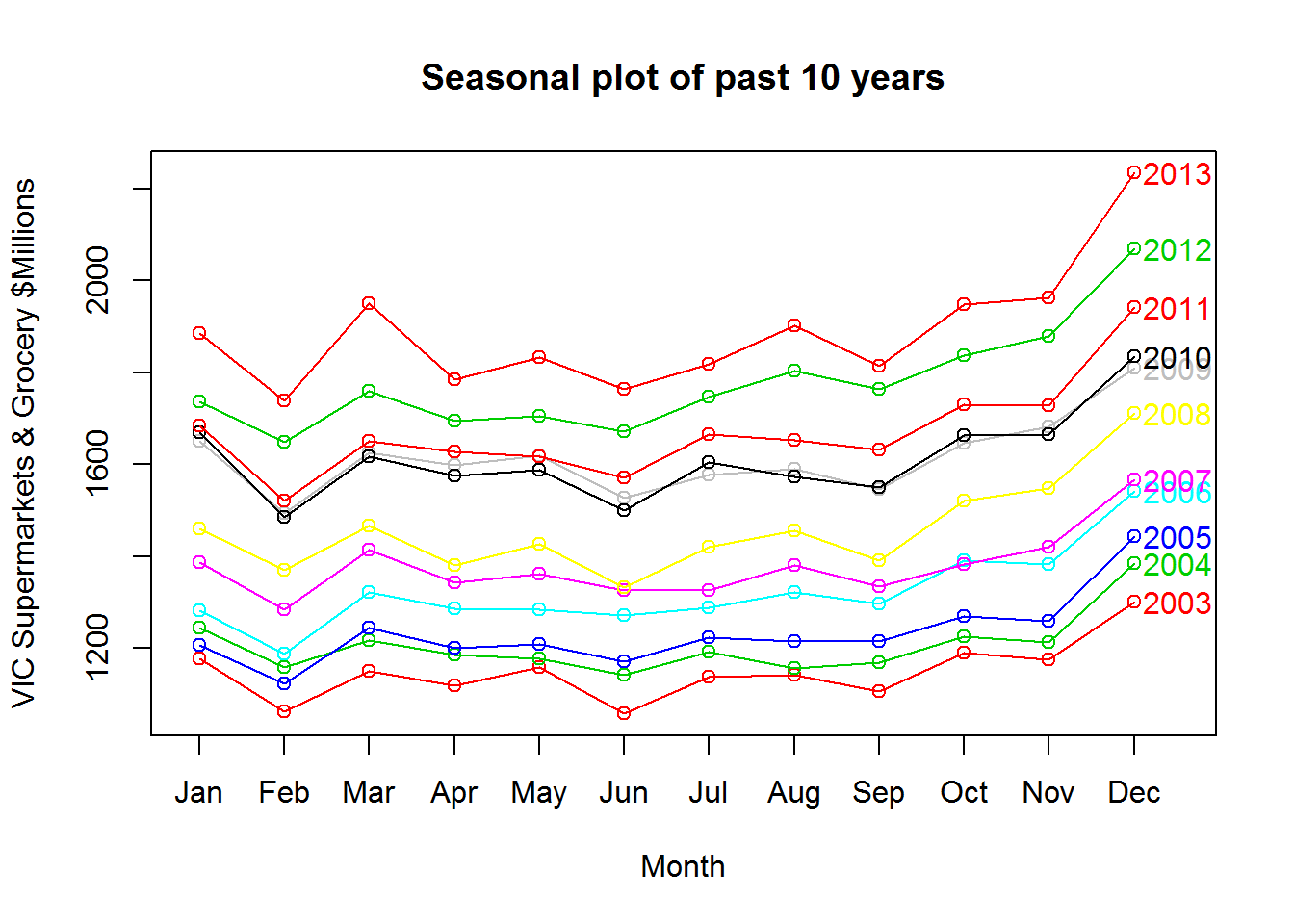Clearly, Victorian supermarket and grocery store turnover is highest in the fourth quarter, during the Christmas period. There appears to be a minor trough mid year and a small spike in March, but apart from Q4, the seasonality is generally small compared to the level of the data. This makes sense, as demand for food is fairly consistent, changing only with population size.

Finally, let’s examine the STL decomposition of the data. We will use a small s.window to allow for changes in seasonality as we have 30 years of data, and we will perform a Box-Cox transformation to determine if the trend is exponential:

``plot(stl(BoxCox(turnover, BoxCox.lambda(turnover)), s.window=7), main="STL decomposition")``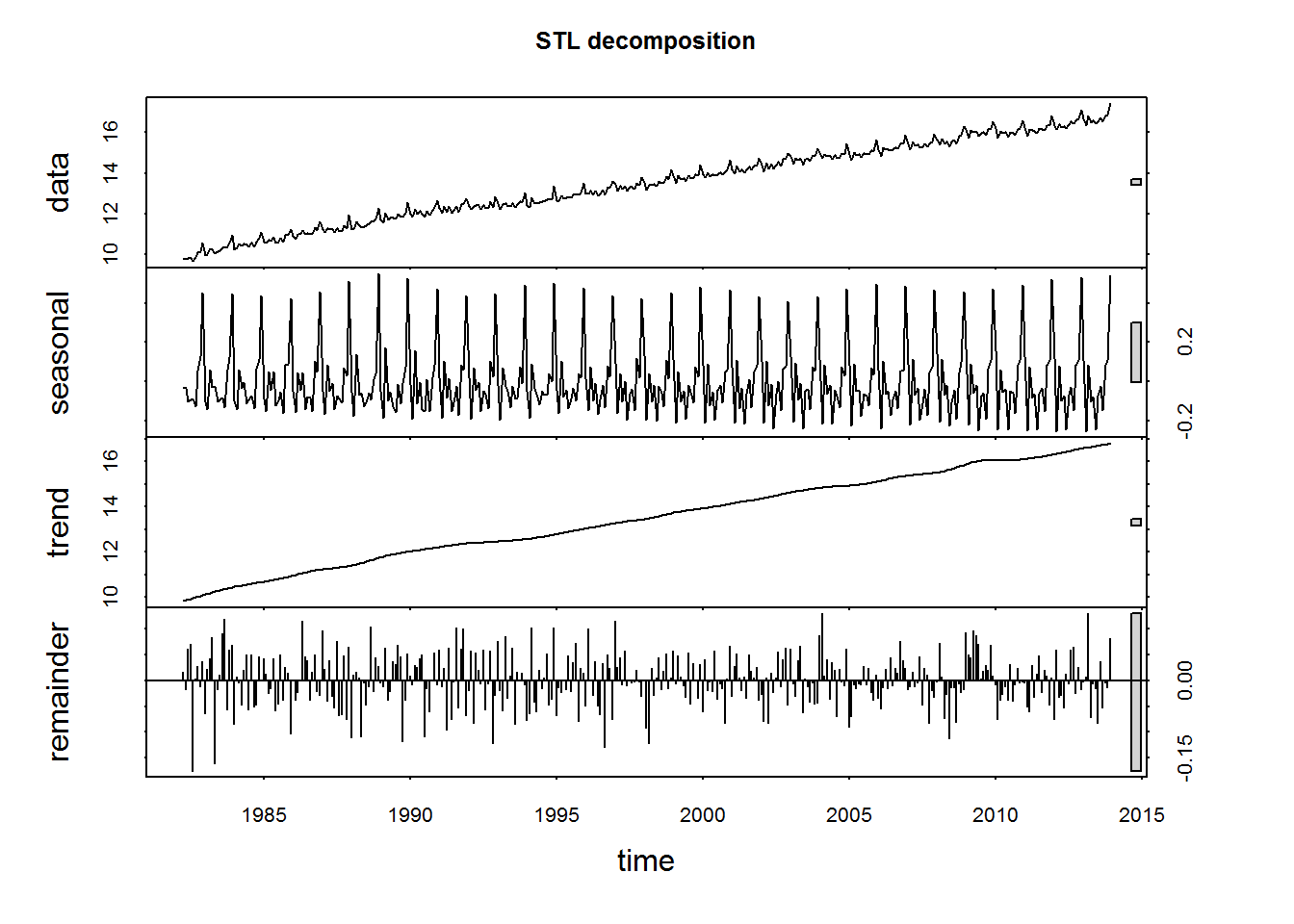This confirms our suspicion that the data is fairly well behaved. Even over 30 years, the seasonal pattern exhibits only small fluctuations. The remainder term is generally small, and there do not appear to be any outliers. The trend appears to be linear, indicating the data is indeed exponentially increasing.

## Transformations and Differencing used

We will keep the Box-Cox transformation of the data we applied in the previous section, as it stabilises the variance of the time series. After testing lambda values by trial and error, `lambda=0.3` was determined to be ideal in minimising variance over time. We will call our transformed time series `transformed_turnover`.

``transformed_turnover <- BoxCox(turnover, 0.3)``

### ETS

As the ETS framework allows for multiplicative terms, we will be fitting ETS models to both the transformed and untransformed series.

### ARIMA

To perform ARIMA modelling on `transformed_turnover`, we need a stationary time series. As the data is trended and exhibits yearly seasonality, let’s try differencing at lag 12: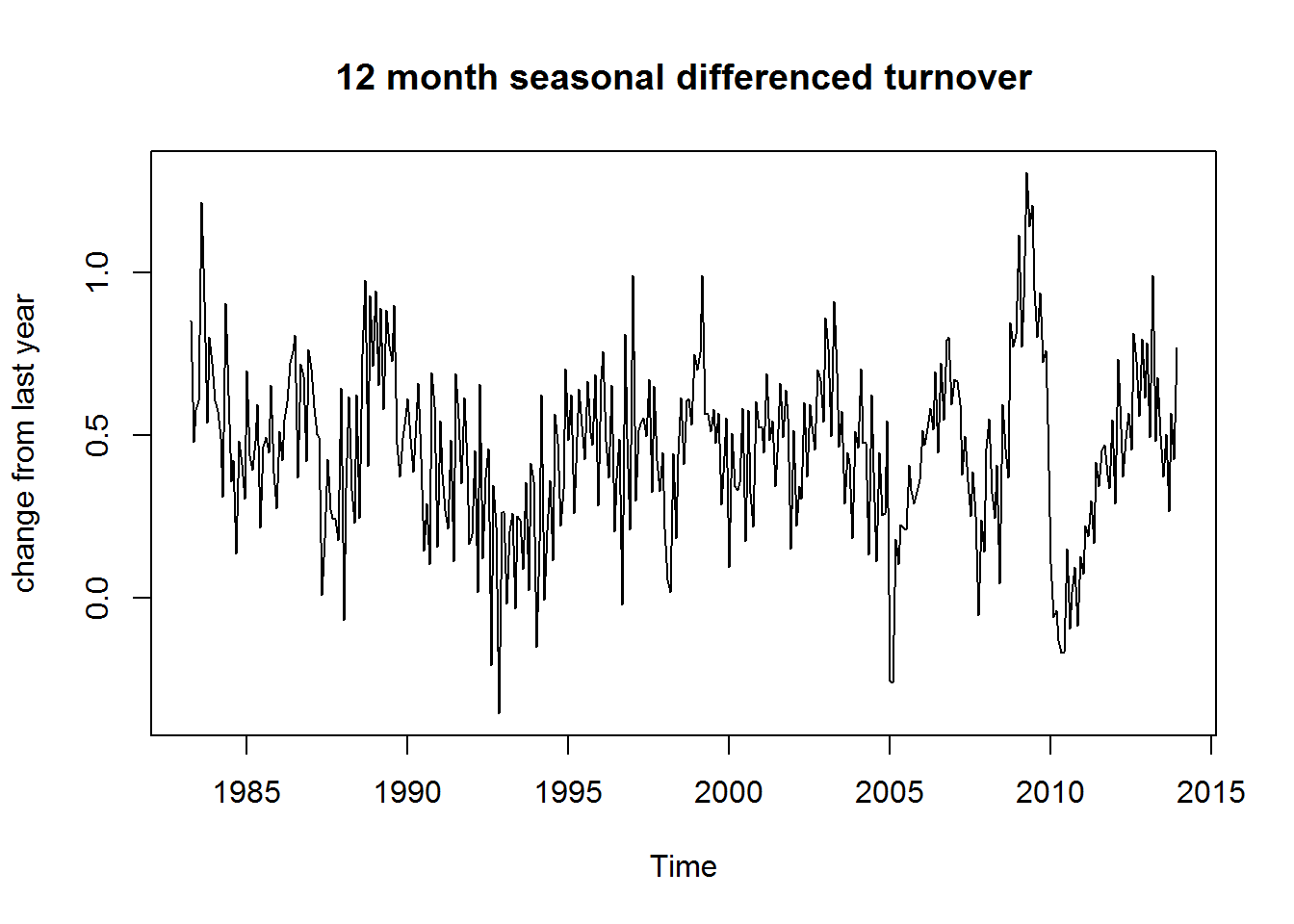The series now appears to be stationary. To confirm this, we can perform a unit root test.

The Augmented Dickey-Fuller test tests against the null hypothesis that the data is non-stationary, so a low p-value will lead us to reject the hypothesis that the data is non-stationary.

``adf.test(diff(transformed_turnover,12),k=12)``
``````## Warning in adf.test(diff(transformed_turnover, 12), k = 12): p-value
## smaller than printed p-value``````
``````##
##  Augmented Dickey-Fuller Test
##
## data:  diff(transformed_turnover, 12)
## Dickey-Fuller = -6.6349, Lag order = 12, p-value = 0.01
## alternative hypothesis: stationary``````

As p-value < 0.01, we reject the null and conclude that we have performed sufficient differencing to achieve stationary data.

Another unit root test we can perform is the Kwiatkowski-Phillips-Schmidt-Shin test, under which the null hypothesis is that the data is stationary.

``kpss.test(diff(transformed_turnover,12))``
``````## Warning in kpss.test(diff(transformed_turnover, 12)): p-value greater than
## printed p-value``````
``````##
##  KPSS Test for Level Stationarity
##
## data:  diff(transformed_turnover, 12)
## KPSS Level = 0.11761, Truncation lag parameter = 4, p-value = 0.1``````

As p-value > 0.1, we cannot reject the null hypothesis that the data is stationary.

Therefore, we need to take a Box-Cox transformation with lambda=0.3 and difference at lag 12 for our data to be suitable for ARIMA modelling.

## Model Shortlist and Methodology

For the purpose of model selection, we need to split our data into a training set and a test set.

``````training = window(turnover, end=c(2011,12))
test = window(turnover, start=c(2012,1))``````

### ETS

As there are only thirty possible ETS models of the form Error = {A, M}, Trend = {N, A, Ad, M, Md}, Seasonal = {N, A, M}, it is feasible for us to estimate all possible models and select based on AICc, log-likelihood and test set performance.

Using `ets()`, we estimate the best ETS model on the untransformed training data by log-likelihood:

``ets_fit <- ets(training)``

On the untransformed data, an ETS(M,Ad,M) model was selected based on the log-likelihood. The same model was selected under the AICc. Examining the accuracy measures:

``accuracy(forecast(ets_fit, h=24), test)``
``````##                     ME     RMSE      MAE       MPE     MAPE      MASE
## Training set  2.290737 21.95484 16.61956 0.2215429 2.097474 0.3317527
## Test set     57.048463 72.76946 60.30324 2.9986990 3.187466 1.2037480
##                     ACF1 Theil's U
## Training set -0.00431359        NA
## Test set      0.39794553 0.6295578``````

We will also estimate an ETS model on the transformed data.

``ets_fit2 <- ets(training, lambda=0.3)``

On the transformed data, an ETS(A,A,A) model was selected based on the log-likelihood. The same model was selected under the AICc. Examining the accuracy measures:

``accuracy(forecast(ets_fit2, h=24), test)``
``````##                      ME     RMSE      MAE         MPE     MAPE      MASE
## Training set -0.1134726 21.26958 16.17207 -0.02434443 2.080682 0.3228201
## Test set     26.2667075 45.99603 34.88606  1.33263468 1.829578 0.6963809
##                     ACF1 Theil's U
## Training set -0.01742633        NA
## Test set      0.15591455 0.3954222``````

As our two models are from two different data sets, we cannot compare their AICc values and some of the accuracy measures. However, the MASE is suitable for comparing the two. The ETS(A,A,A) model on the transformed data performed slightly better than the ETS(M,Ad,M) model on the untransformed data, and significantly better on the test set.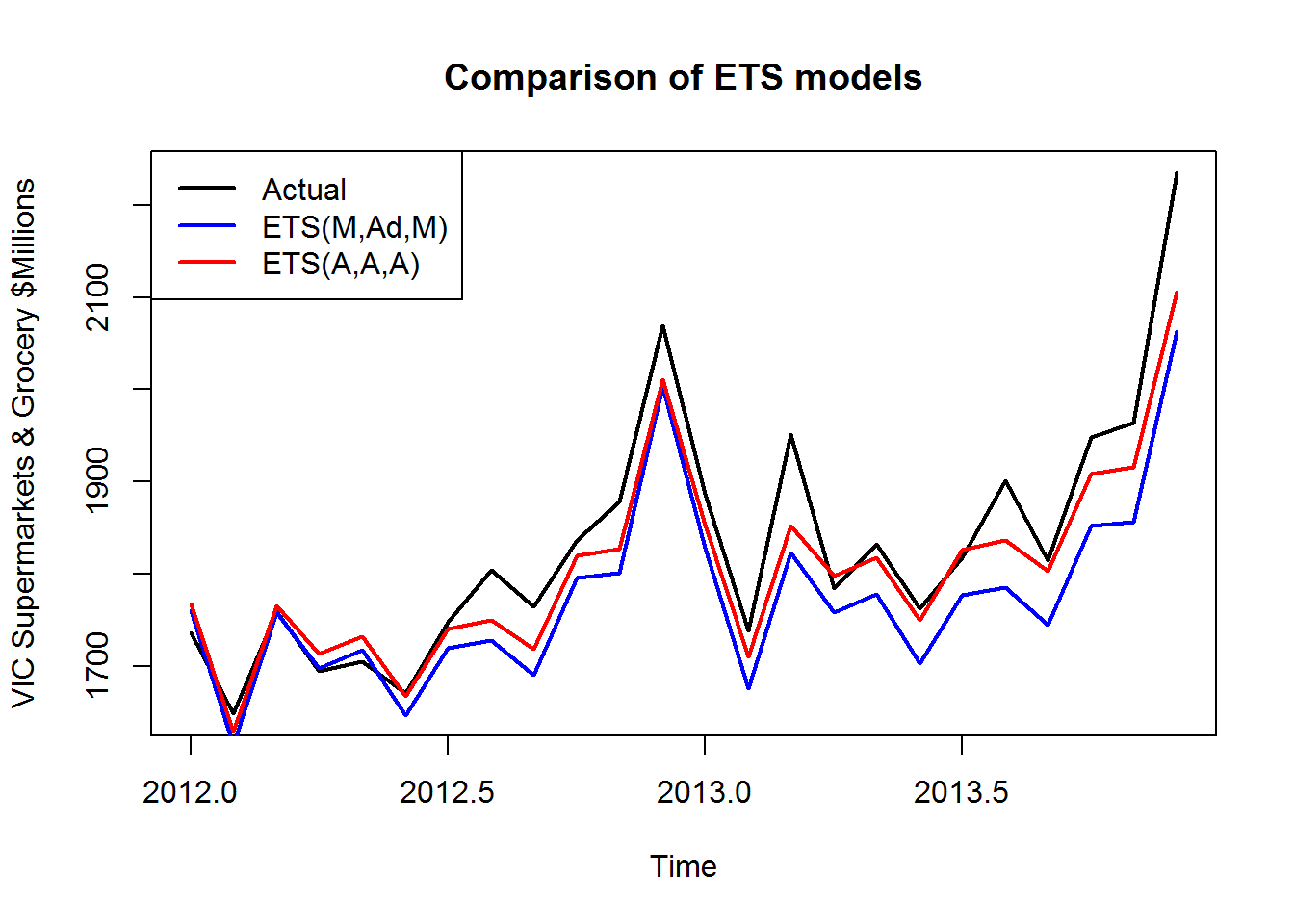It appears that both models underestimated the test set, but the ETS(A,A,A) model on the transformed data performed slightly better overall.

To confirm this result, we can once again estimate ETS models on the transformed and untransformed data, this time on the full data set. Once again, the model fitted to the untransformed data was the ETS(M,Ad,M) model, and the model fitted to the transformed data was the ETS(A,A,A) model. Comparing the one-step forecast accuracy of these models:

``accuracy(ets(turnover))``
``````##                    ME     RMSE      MAE       MPE     MAPE      MASE
## Training set 2.870866 22.72774 17.16524 0.2634732 2.049015 0.3183573
##                     ACF1
## Training set -0.03063171``````
``accuracy(ets(turnover, lambda=0.3))``
``````##                     ME     RMSE     MAE         MPE     MAPE      MASE
## Training set 0.1902218 22.36463 16.9889 -0.05128769 2.050136 0.3150867
##                     ACF1
## Training set -0.04322947``````

Once again, the ETS(A,A,A) model performs slightly better under the MASE, so we will use this as our ETS model.

### ARIMA

We can gain some insight into what ARIMA models may be appropriate by looking at the ACF and PACF plots.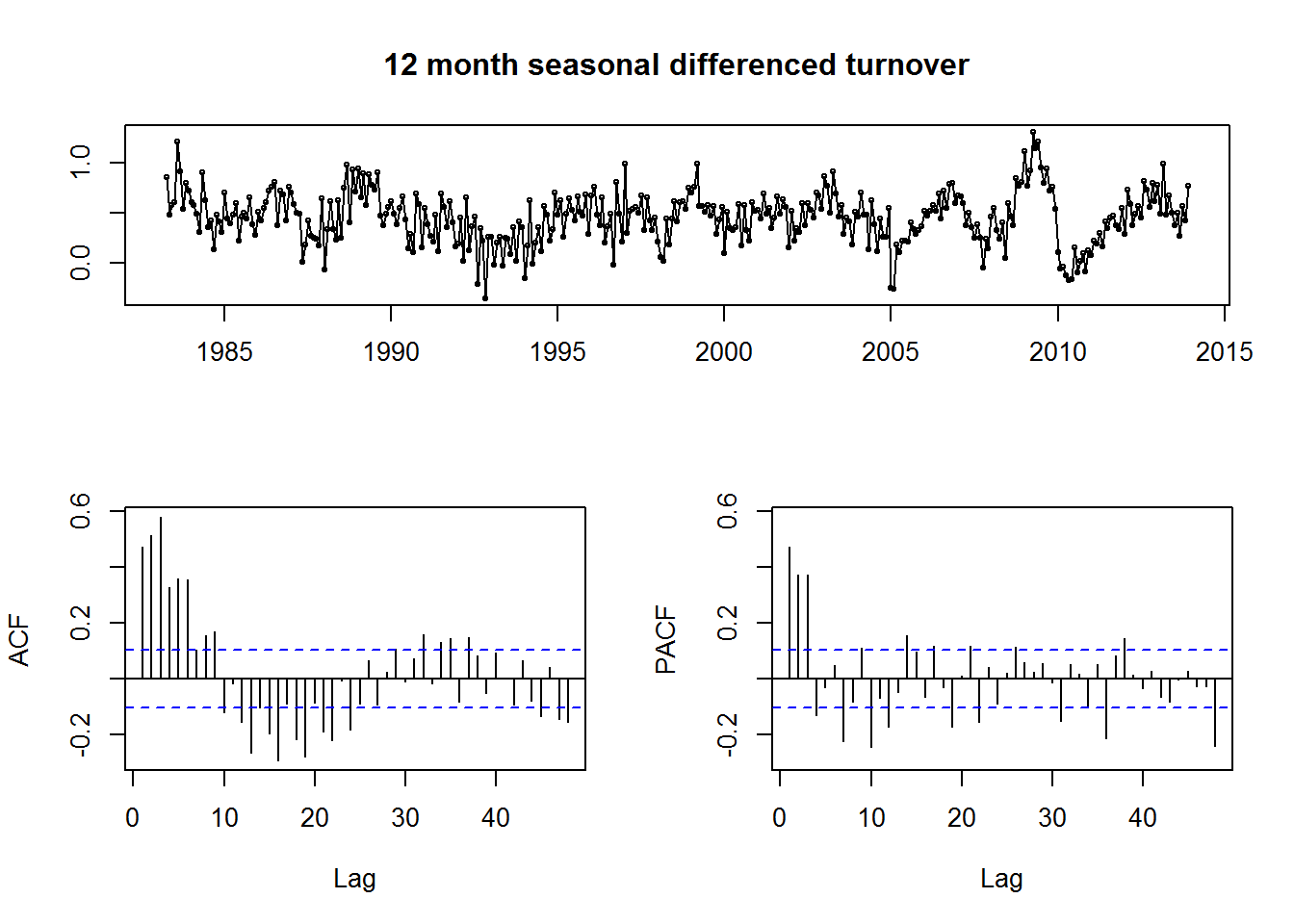The key feature of the ACF plot is that it forms an exponentially decaying sinusoid.

Interestingly, the plot appears to be grouped into groups of three lags. This may suggest that the data exhibits a second, weaker seasonality with quarterly frequency. However, we cannot explore this possibility further as our ARIMA models will only consider one degree of seasonality.

The key feature of the PACF plot is the three large spikes at lags 1, 2 and 3. The PACF plot also features spikes at lags 12, 24, 36 and 48.

Unfortunately, there are still a number of significant lags in the PACF plot that cannot be accounted for, even after trialling various combinations of up to two differences, and up to two seasonal differences. This may be due to the suspected sub-seasonal patterns in the data.

Despite this, the ACF and PACF give some insight into our potential ARIMA models. There is no simple ARIMA model suggested by the shape of the ACF and PACF, so we will explore individual terms.

The sinusoidal decay of the ACF and three spikes at the non-seasonal lags 1, 2 and 3 on the PACF suggest an AR(3) term.

The spikes at lags 12, 24, 36 and 48 are not matched on the ACF, suggesting we should include a seasonal AR(4) term. However, we should also explore lower order seasonal AR terms, as higher order terms are likely to increase the AIC.

Having determined a possible model to be ARIMA(3,0,0)(4,1,0)12, we will explore all variations of the form ARIMA(3,0,x)(y,1,z)12, where x = 0, 1 or 2; y = 0, 1, 2, 3 or 4; and z = 0, 1 or 2, with and without drift. The following code returns a matrix of AICc and accuracy measures, and takes approximately 20 minutes to execute.

``````training <- window(turnover, end=c(2011,12))
test <- window(turnover, start=c(2012,1))
arima_model <- function(){
output = matrix(nrow=0, ncol=13)
for (x in 0:2){
for (y in 0:4){
for (z in 0:2){
for (w in 0:1){
fit <- Arima(training, lambda=0.3, order=c(3,0,x), seasonal=c(y,1,z), include.drift = w)
output = rbind(output, c(fit\$aicc,x,y,z,w,accuracy(forecast(fit, h=24), test)[2,]))
print(output)
}
}
}
}
return(output)
}
out <- arima_model()
which.min(out[,1])  # returns model number with minimum AICc
for (i in 6:13){which.min(out[,1])} returns model numbers with test set accuracy measures``````

Of the 90 models tested, the following four had an AICc < -300, or was the best in one of the training measures. For comparison, the fitted model from `auto.arima(training, lambda=0.3, stepwise=FALSE, approximation=FALSE)` is included.

Model AICc ME RMSE MAE MPE MAPE MASE
ARIMA(3,0,0)(4,1,2)12 with drift -303.57 20.61 33.38 25.20 1.08 1.33 0.50
ARIMA(3,0,1)(4,1,2)12 with drift -302.10 20.66 33.37 25.23 1.08 1.33 0.50
ARIMA(3,0,0)(3,1,2)12 with drift -300.01 25.86 36.58 28.31 1.37 1.51 0.57
ARIMA(3,0,0)(2,1,2)12 with drift -258.25 2.07 31.94 26.44 0.03 1.42 0.53
ARIMA(3,0,0)(0,1,2)12 with drift (auto.arima) -228.03 15.84 39.59 29.70 0.77 1.56 0.59

Based on the AICc, we were correct to assume an AR(4) term would be included in the best model. Though this set of models took a significant amount of time to calculate, 40 of 90 models calculated had an AICc that improved on the best output of `auto.arima()`.

To confirm the result from the training set, I calculated the AICc of the 90 models under consideration, this time with the full data set. Once again, the ARIMA(3,0,0)(4,1,2)12 with drift had the lowest AICc = -329.39.

## Selected Models

### ETS

Based on the AICc and the log-likelihood, the ETS(M,Ad,M) model performed best on the untransformed data, and the ETS(A,A,A) model performed best on the transformed data.

I then compared the forecasts of these two models using a training set and a test set, and the one-step forecasts of these models trained on the full data set. The ETS(A,A,A) model on the transformed data performed slightly better on both of these tests, so I have selected it as my ETS model.

On the training set, the ETS model parameters are as follows:

``````## ETS(A,A,A)
##
## Call:
##  ets(y = training, lambda = 0.3)
##
##   Box-Cox transformation: lambda= 0.3
##
##   Smoothing parameters:
##     alpha = 0.2718
##     beta  = 1e-04
##     gamma = 0.1515
##
##   Initial states:
##     l = 14.3167
##     b = 0.0377
##     s=0.1421 -0.289 -0.0272 0.9429 0.1617 0.0569
##            -0.2495 -0.049 -0.2116 -0.3196 -0.104 -0.0538
##
##   sigma:  0.1861
##
##      AIC     AICc      BIC
## 929.9238 931.5238 991.9676``````

We will perform a set of diagnostic tests on the estimated model to determine if there is room for further improvement. We are looking for uncorrelated residuals, and zero mean.

First, let’s look at a histogram of the residuals.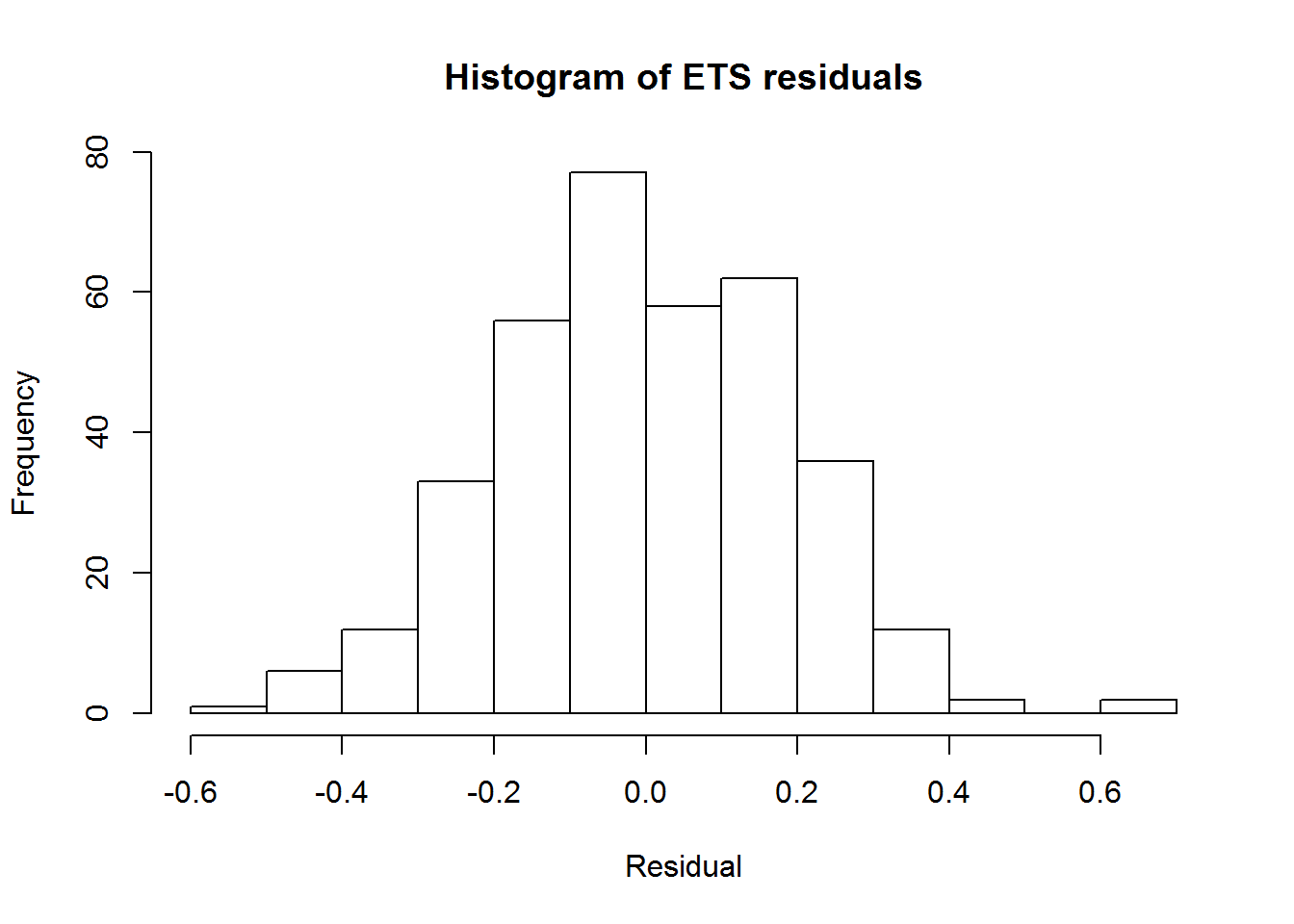The histogram appears to approximate a normal distribution with mean zero, indicating our forecasts are not biased.

Now, let’s perform a Ljung-Box test on the residuals. The null hypothesis of the Ljung-Box test is that the residuals are uncorrelated. We set the number of lags to twice the season length, and the degrees of fit of the model to the number of parameters in the model.

``Box.test(ets_residuals, lag=24, fitdf=16, type="L")``
``````##
##  Box-Ljung test
##
## data:  ets_residuals
## X-squared = 486.67, df = 8, p-value < 2.2e-16``````

Unfortunately, we can be almost certain that the residuals are correlated, based on the extremely low p-value of the Ljung-Box test.

To investigate, let’s look at the PACF and PACF of the residuals: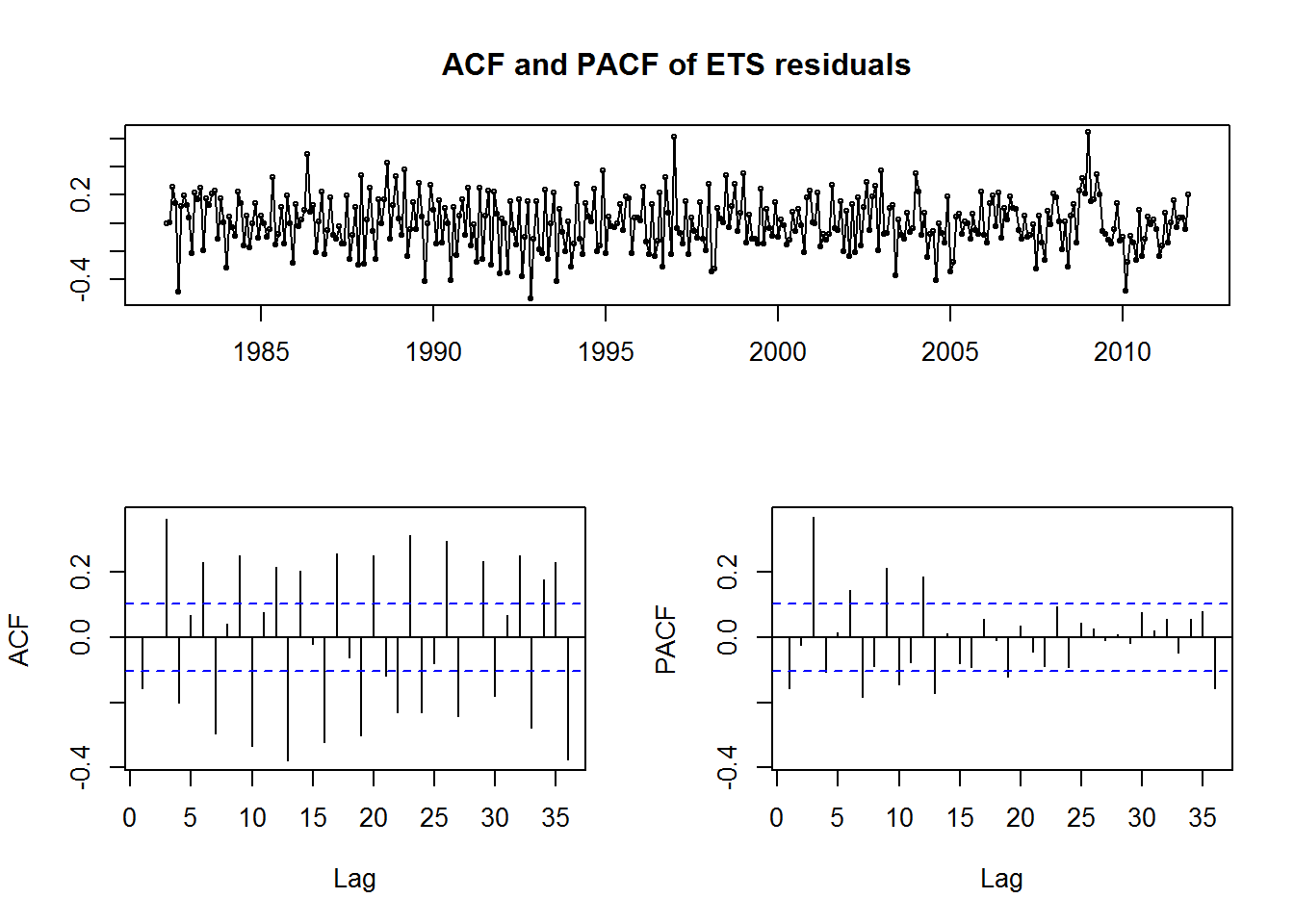It appears our suspicions about a quarterly sub-seasonal pattern were accurate. There is a large spike on the PACF at lag three, and there remains a large degree of autocorrelation in the residuals on the ACF. Unfortunately there is not much we can do to extract this residual information with just an ETS model.

However, we can be confident that the residuals are unbiased, as the mean of the residuals is -0.0001, compared to a mean of the training set of 861. Our forecasts are therefore likely to be unbiased.

Now we will inspect the prediction intervals of the forecast on the test set.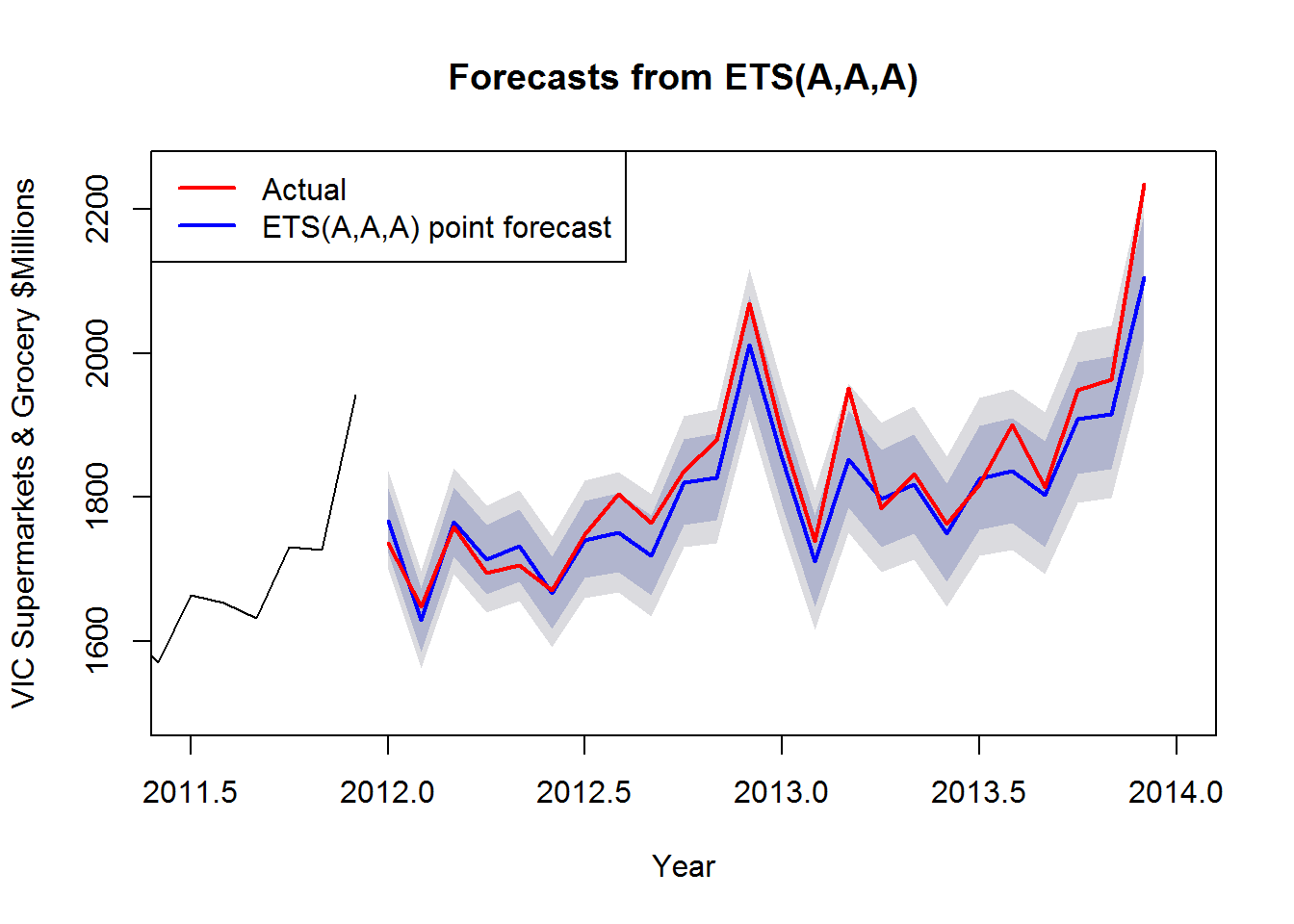``sum(ets_forecast\$upper[,1] < test) + sum(ets_forecast\$lower[,1] > test)``
``##  2``

The 80% prediction intervals appear to be performing well on the test set. Out of 24 predictions, we expect 4.8 observations to lie outside of the 80% prediction intervals. On the test set, 2 actual values were outside the 80% PIs.

### ARIMA

Based on the AICc, the ARIMA(3,0,0)(4,1,2)12 with drift is the best ARIMA model for the data set. While one of the other models under consideration had the best ME, RMSE and MPE, the ME and MPE suffer from cancellation of positive and negative errors, and the RMSE was almost identical to that of the selected model, so we can safely discard it.

On the training set, the ARIMA model parameters are as follows:

``````arima_model <- Arima(training, lambda=0.3, order=c(3,0,0), seasonal = c(4,1,2), include.drift=TRUE)
arima_model``````
``````## Series: training
## ARIMA(3,0,0)(4,1,2) with drift
## Box Cox transformation: lambda= 0.3
##
## Coefficients:
##          ar1     ar2     ar3    sar1     sar2     sar3     sar4     sma1
##       0.3292  0.3053  0.2444  0.3909  -0.6227  -0.3760  -0.1712  -1.0510
## s.e.  0.0538  0.0531  0.0550  0.0971   0.0596   0.0591   0.0737   0.0938
##         sma2   drift
##       0.8375  0.0377
## s.e.  0.1075  0.0023
##
## sigma^2 estimated as 0.02002:  log likelihood=163.18
## AIC=-304.37   AICc=-303.57   BIC=-262.09``````

Once again, we will examine the residuals of the model to see if they are uncorrelated with zero mean.

``arima_residuals <- residuals(arima_model)``

First, let’s look at a histogram of the residuals.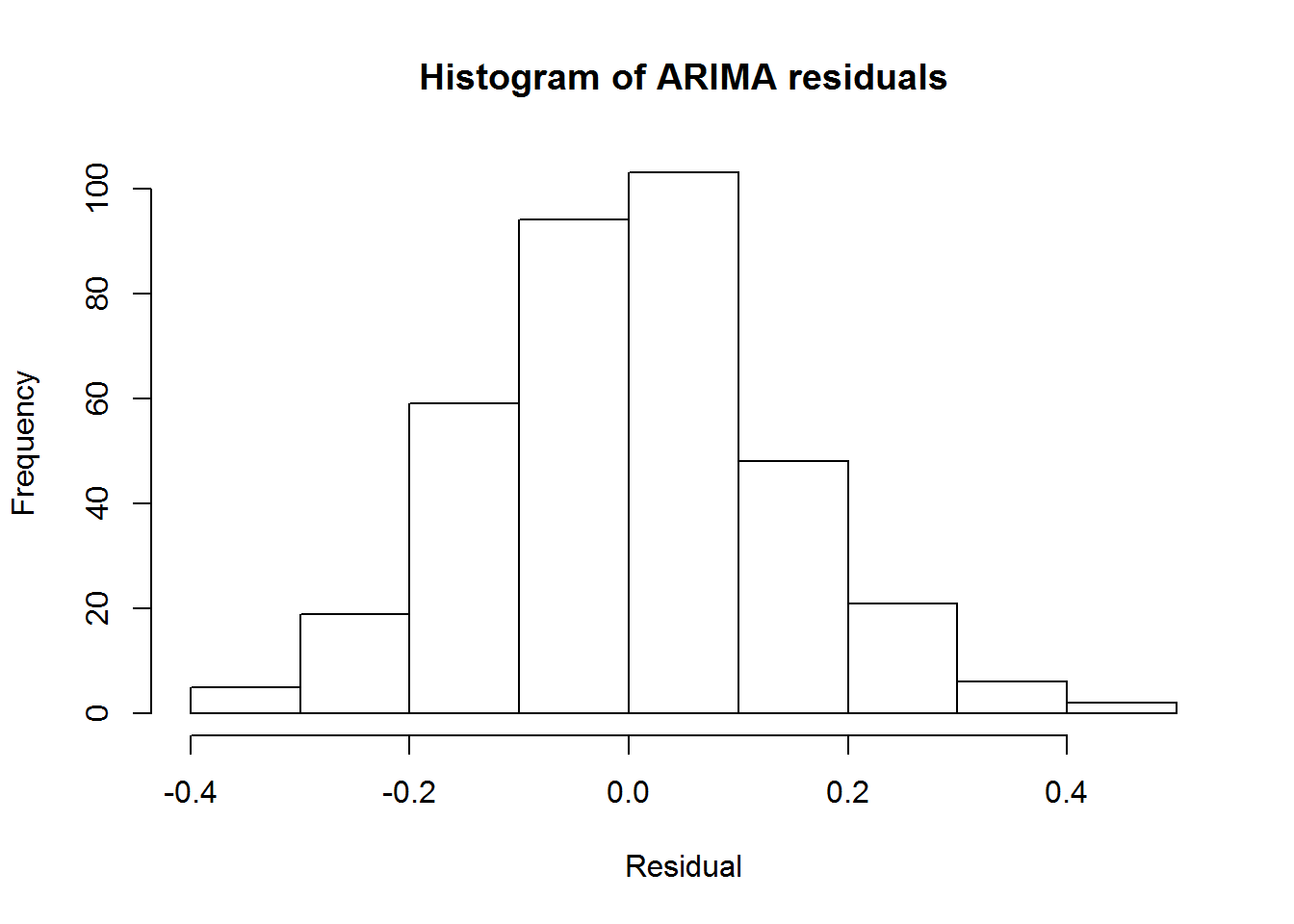Once again, the histogram appears to approximate a normal distribution with mean zero, indicating our forecasts are not biased.

Performing the Ljung-Box test:

``Box.test(arima_residuals, lag=24, fitdf=10, type="L")``
``````##
##  Box-Ljung test
##
## data:  arima_residuals
## X-squared = 25.136, df = 14, p-value = 0.03325``````

Our Arima model performs much better on the Ljung-Box test, but still fails with p=value = 0.03325. This better performance is likely due to the relatively high order of the seasonal term P = 4.

Examining the ACF and PACF of the residuals: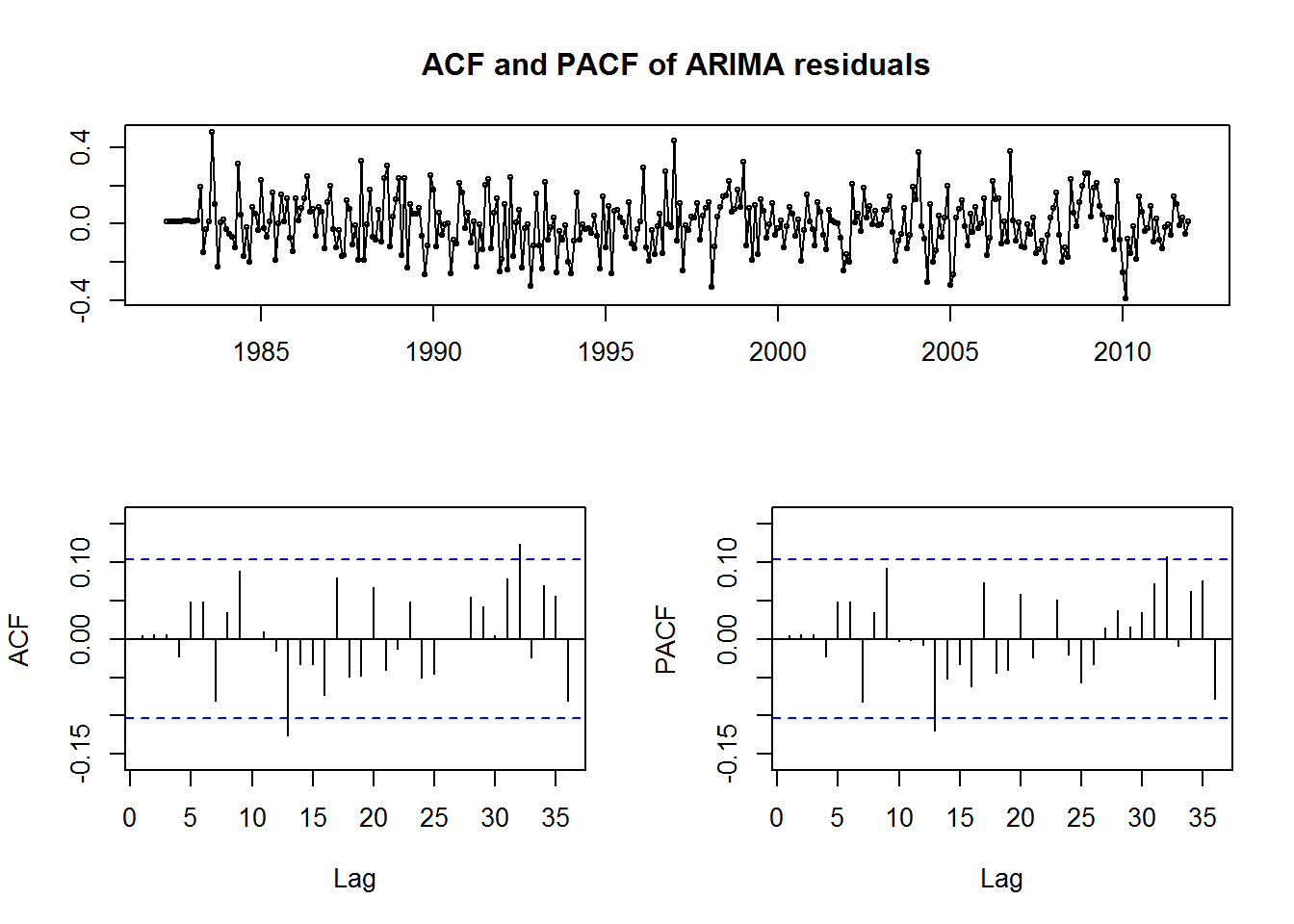The better performance of the ARIMA model is confirmed in the ACF and PACF plots. only two lags exceed the significance level in both, which is approximately what we would expect for 36 lags at 95% significance. Based on these diagnostics, our ARIMA model is likely to produce better forecasts than our ETS model.

Now we will inspect the prediction intervals of the ARIMA forecast.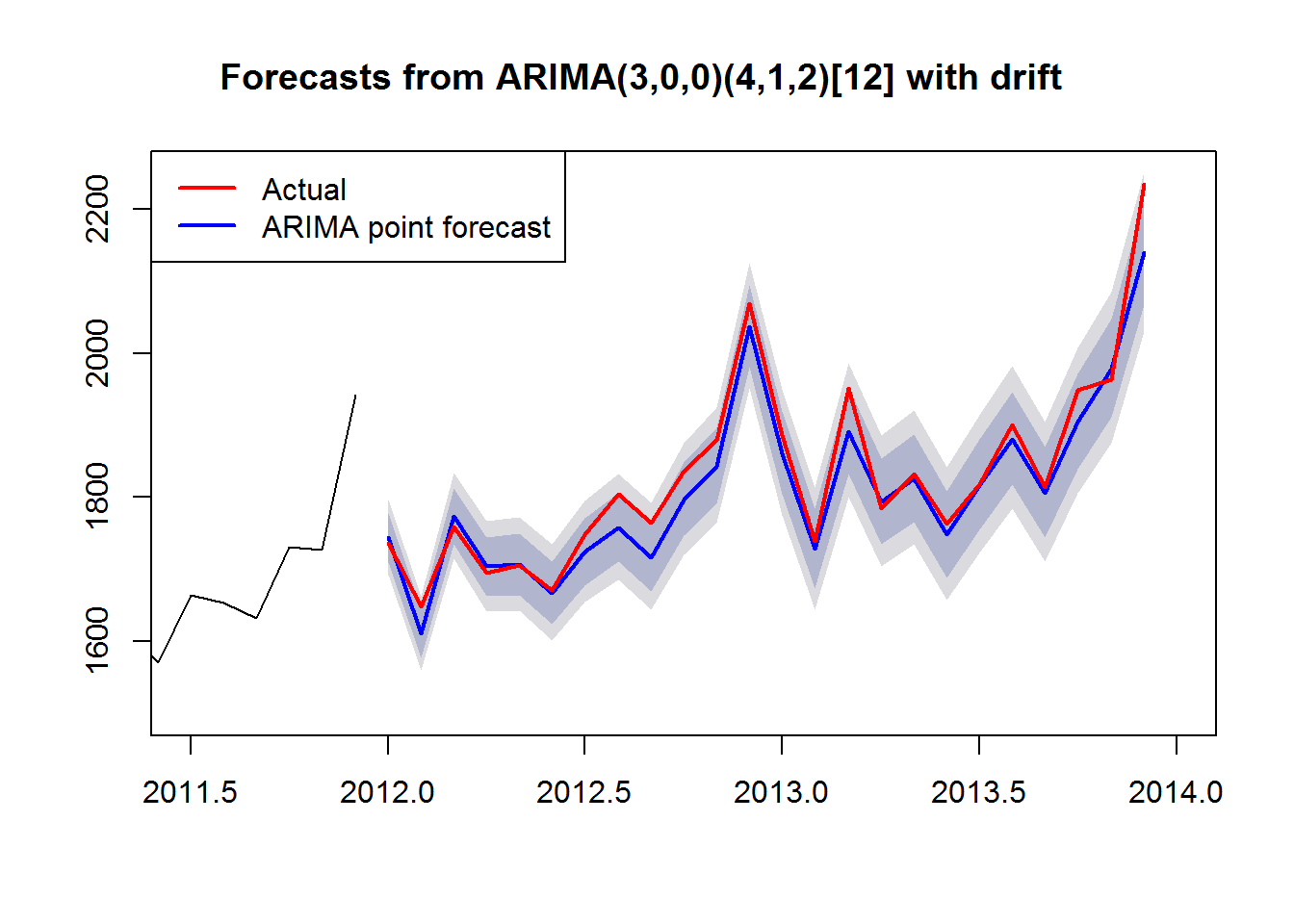``sum(arima_forecast\$upper[,1] < test) + sum(arima_forecast\$lower[,1] > test)``
``##  2``

Once again, the 80% prediction intervals appear to be performing well on the test set. Out of 24 predictions, we expect 4.8 observations to lie outside of the 80% prediction intervals. On the test set, 2 actual values were outside the 80% PIs.

## Comparison of Results

Having established that the ARIMA model is likely to perform better than the ETS model based on the residual diagnostics, we will now compare the diagnostics from the test set.

``````ets_accuracy <- accuracy(ets_forecast, test)
arima_accuracy <- accuracy(arima_forecast, test)
ets_accuracy``````
``````##                      ME     RMSE      MAE         MPE     MAPE      MASE
## Training set -0.1134726 21.26958 16.17207 -0.02434443 2.080682 0.3228201
## Test set     26.2667075 45.99603 34.88606  1.33263468 1.829578 0.6963809
##                     ACF1 Theil's U
## Training set -0.01742633        NA
## Test set      0.15591455 0.3954222``````
``arima_accuracy``
``````##                       ME     RMSE      MAE          MPE     MAPE      MASE
## Training set -0.05125758 16.09253 11.90924 -0.006155457 1.490384 0.2377272
## Test set     20.60624049 33.38279 25.20328  1.076823700 1.332528 0.5030973
##                    ACF1 Theil's U
## Training set  0.1134248        NA
## Test set     -0.1192096  0.291364``````
``abs(arima_accuracy) < abs(ets_accuracy)``
``````##                ME RMSE  MAE  MPE MAPE MASE  ACF1 Theil's U
## Training set TRUE TRUE TRUE TRUE TRUE TRUE FALSE        NA
## Test set     TRUE TRUE TRUE TRUE TRUE TRUE  TRUE      TRUE``````

As expected, the ARIMA model performs better than the ETS model on almost all metrics of forecast accuracy on the test set. This bears out in the plot: